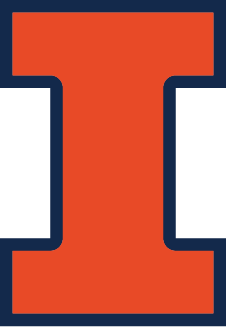CS 440/ECE 448
Margaret Fleck

## A* Search 1Shakey the robot (1966-72), from Wired Magazine

## $$A^*$$ search

$$A^*$$ search was developed around 1968 as part of the Shakey project at Stanford. It's still a critical component of many AI system.s The Shakey project ran from 1966 to 1972. Here's some videos showing Shakey in action.

Towards the end of the project, it was using a PDP-10 with about 800K bytes of RAM. The programs occupied 1.35M of disk space. This kind of memory starvation was typical of the time. For example, the guidance computer for Apollo 11 (first moon landing) in 1969 had only 70K bytes of storage.

## The Problem

Cost so far is a bad predictor of how quickly a route will reach a goal. If you play around with this search technique demo by Xueqiao Xu, you can see that BFS and UCS ("Dijkstra") search a widening circular space, most of which is in the direction opposite to the goal. When they assess states, they consider only distance from the starting position, but not the distance to reach the goal.

## Recall UCS

Recall that uniform-cost search(UCS) has three types of states:

• unseen
• done/closed = neighbors all explored, allegedly finished with this state
• open/frontier = seen but neighbors need to be explored

The frontier is stored in a priority queue, with states ordered by their distance g(s) from the start state. At each step of the search, we extract the state S with lowest g value. For each of S's neighbors, we check if it has already been seen. If it's new, add it to the frontier.

Whe we return to a previously-seen state S via a new, shorter path, we update the stored distance g(S) and S's position in the priority queue.

Search stops when the goal reaches the top of the queue (not when the goal is first found).

## $$A^*$$ search

The idea behind $$A^*$$ search is use a better estimate of how promising each state is. Specifically, we sum

• cost so far, g(x), and
• estimated ("heuristic") distance to goal, h(x).

So $$A^*$$ search is essentially the UCS algorithm but prioritizing states based on f(x) = g(x) + h(x) rather than just g(x).

With a reasonable choice of heuristic, $$A^*$$ does a better job of directing its attention towards the goal. Try the search demo again, but select $$A^*$$.